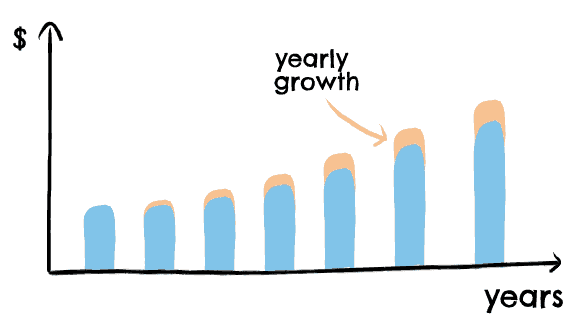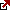# What is Perpetuity Growth Rate? – Terminal Growth Rate Calculation

Business projection is one of the most important things. Long-term traders need to know to create reasonable forecasts for company stocks. The terminal growth rate is one of the essential criteria in that process.

## What is the terminal growth rate?

Perpetual growth rate, or terminal growth rate, is the rate at which a company’s earnings or cash flows are expected to grow indefinitely. It is a fundamental assumption used in financial modeling, especially in valuation models such as the discounted cash flow (DCF) method.The perpetual growth rate is based on the premise that a company will continue to generate earnings or cash flows at a steady rate and that this rate will be sustainable over the long term. It is typically expressed as a percentage and can be estimated using various methods, such as historical growth rates, industry benchmarks, or analyst forecasts.

In a DCF analysis, the perpetual growth rate estimates the value of a company’s future cash flows beyond a certain period (usually 5-10 years), known as the forecast period. This is done by applying a terminal value formula to the cash flows generated in the forecast period, assuming they will continue to grow at the perpetual growth rate. The terminal value is then discounted back to its present value using a discount rate, which reflects the risk associated with the investment.

The perpetual growth rate is an essential input in financial modeling, as it can significantly impact a company’s estimated value. However, it is also a highly uncertain assumption, as predicting how a company’s earnings or cash flows will evolve over the long term is difficult. As such, it is essential to use a range of growth rate scenarios and sensitivity analyses to test the robustness of the valuation model.

The terminal growth rate is the constant rate, estimation of a business’s performance over the expected future revenues. Simply put, the terminal growth rate is the firm’s expected free cash flows that are assumed to grow forever.

To simplify, these are terminal growth rate characteristics:

• The perpetual growth rate is when a company’s earnings or cash flows are expected to grow indefinitely.
• It is also known as the terminal growth rate.
• It is used in financial modeling, especially in valuation models such as the discounted cash flow (DCF) method.
• The perpetual growth rate is based on the premise that a company will continue to generate earnings or cash flows at a steady rate and that this rate will be sustainable over the long term.
• It is typically expressed as a percentage and can be estimated using various methods, such as historical growth rates, industry benchmarks, or analyst forecasts.
• In a DCF analysis, the perpetual growth rate estimates the value of a company’s future cash flows beyond a certain period (usually 5-10 years), known as the forecast period.
• This is done by applying a terminal value formula to the cash flows generated in the forecast period, assuming they will continue to grow at the perpetual growth rate.
• The terminal value is then discounted back to its present value using a discount rate, which reflects the risk associated with the investment.
• The perpetual growth rate is an essential input in financial modeling, as it can significantly impact a company’s estimated value.
• However, it is also a highly uncertain assumption, as predicting how a company’s earnings or cash flows will evolve over the long term is challenging.
• As such, it is essential to use a range of growth rate scenarios and sensitivity analyses to test the robustness of the valuation model.

This rate is a fixed rate in which an entity is intended to expand regardless of its projected unrestricted cash revenues. The terminal growth rate would continue to increase constantly instead of predicting available cash revenues for each cycle beyond the market growth. A retailer can expect the end of the market growth to the perpetuity growth rate in this growth rate. This article will clearly explain the working and operation of the terminal rate.

## How to calculate the Perpetuity growth rate?

Before moving forward, let us explain the definition of the perpetuity growth rate. Perpetuity growth rate represents the calculation of a firm’s 10th year’s income and is determined by the difference in capital costs and the growth rate plus the firm’s long-term rate.

Perpetuity growth rate, also known as constant growth rate or terminal growth rate, is the rate at which a company’s cash flows are expected to grow indefinitely after a certain period. It is used in financial modeling to calculate the present value of perpetuity or to estimate the terminal value of a company’s future cash flows.

Steps to calculate the perpetuity growth rate:

• Determine the current or starting cash flow of the perpetuity. This could be a dividend, bond coupon, or any other recurring cash flow.
• Determine the expected growth rate of the cash flow for a certain period, usually 5-10 years. This could be based on historical growth rates, industry benchmarks, or analyst forecasts.
• Calculate the perpetuity cash flow beyond the growth period by using the formula: Perpetuity Cash Flow = Cash Flow x (1 + Growth Rate) / (Discount Rate – Growth Rate)
• The perpetuity growth rate is when the cash flows beyond the growth period are expected to grow indefinitely. This can be calculated by rearranging the formula above: Growth Rate = Discount Rate – Perpetuity Cash Flow / Cash Flow.

## Perpetuity growth rate calculation Example

Assume a company has a current cash flow of \$100, and it is expected to grow at a rate of 5% for the next ten years. The discount rate is 10%.

To calculate the perpetuity growth rate beyond the ten years, we first need to calculate the perpetuity cash flow as follows:

Perpetuity Cash Flow = \$100 x (1 + 5%) / (10% – 5%) = \$2,000

Then, we can calculate the perpetuity growth rate as follows:

Perpetuity Growth Rate = 10% – \$2,000 / \$100 = -90%

Note that a negative perpetuity growth rate implies that the cash flows are expected to decline over time, which may not be a realistic assumption. In practice, perpetuity growth rates are usually favorable but conservative to account for the uncertainty of long-term growth rates.

The terminal rate predicts the business’s continued growth (or decline) at a constant and consistent pace. This growth rate is expected to produce a steady result if the terminal rate of the firm changes, and multiple-phase terminal costs should be measured at stages. Nevertheless, if the growth rate remains negative (or decreases), the business is expected to collapse and break shortly.

In general, perpetual growth rates vary from the average inflation of 3% – 4% to the historic GDP rate of 5% – 6%. If the constant rate exceeds 7%, its growth will surpass economic growth. The projected terminal growth rate and the degree to which the business reaches a steady state are always measured significantly.

It is standard practice to conclude that there will be different growth trends for forecasts of free cash revenue in business according to the phase of the economic cycle in which a company currently works.

We expect high growth rates for a company in its preliminary development stage (usually over 10%). This is because the business has built its role in the company and aims to grow its market share. As such, sales and free cash flow should increase rapidly.

A comparatively decelerated development period follows the rapid growth process since it will probably struggle to keep its high growth level due to increasing market rivalry. As a result, the company will continue to expand, but not at its previous significant growth level. Nevertheless, the company is expected to retain a stable market share and profits as it is nearer to maturity.

We predict that the market will develop at a mature stage at the terminal growth rate. A terminal growth rate higher than the average GDP value implies that its output is indefinitely faster than in the market.

## Perpetuity Growth Rate Limitations

Here are some limitations of the Perpetuity Growth Rate:

1. Uncertainty: The perpetuity growth rate is based on the assumption that a company’s cash flows will continue to grow indefinitely at a constant rate. However, this assumption is highly uncertain and difficult to predict, especially over the long term.
2. Sensitivity to inputs: The perpetuity growth rate is a critical input in financial modeling, and changes in the growth rate can significantly impact a company’s estimated value. As such, testing the model’s sensitivity to different growth rate scenarios and other inputs is essential.
3. Limited usefulness for young or growing companies: The perpetuity growth rate is most useful for mature companies with stable cash flows and a long operating history. For young or growing companies, the perpetuity growth rate may not be a meaningful or realistic assumption.
4. Risk of overvaluing or undervaluing companies: If the perpetuity growth rate is too high, it can result in an overvaluation of the company’s future cash flows, leading to an overvalued stock price. On the other hand, if the perpetuity growth rate is too low, it can undervalue the company’s future cash flows, leading to an undervalued stock price.
5. Dependence on discount rate: The perpetuity growth rate is calculated based on the discount rate used in the valuation model. Changes in the discount rate can also significantly impact the estimated value of a company. As such, choosing an appropriate discount rate that reflects the risk associated with the investment is essential.

## Conclusion

As we saw earlier, the terminal growth rate is utilized while estimating a company’s end value. A multi-stage method, like the terminal growth rate, reduces a company’s cash flow. It also analyzes the estimated business’s marginal valuation, an essential step for, therefore, cothereforthereforeher.

Latest posts by Fxigor (see all)

Fxigor

Igor has been a trader since 2007. Currently, Igor works for several prop trading companies. He is an expert in financial niche, long-term trading, and weekly technical levels. The primary field of Igor's research is the application of machine learning in algorithmic trading. Education: Computer Engineering and Ph.D. in machine learning. Igor regularly publishes trading-related videos on the Fxigor Youtube channel. To contact Igor write on: igor@forex.in.rs

Trade gold and silver. Visit the broker's page and start trading high liquidity spot metals - the most traded instruments in the world.Diversify your savings with a gold IRA.

Gold & silver portfolio-building assistance from product professionals.

If you want to trade stocks try: